# Iterative Tower of Hanoi

## Introduction

Tower of Hanoi is a famous game or puzzle consisting of three rods with some disks of various sizes in which we have to shift the disks from one rod to another to get arranged in ascending order. There will be some conditions which we need to follow to place the disks in a particular order.

The Tower of Hanoi problem can be solved using the Recursive method, which is better than the iterative one. We will discuss the conditions and the code to solve the iterative solution of the Tower of Hanoi.

Without any further delays, let’s move on to the problem statement.

## Problem Statement

We will be given three rods and ‘n’ number of disks piled on top of each other. Disks are arranged to end up in ascending order, i.e., the disk having the smallest diameter on the top.

Objective: The objective of this puzzle is to move all the disks from one pole(source pole) to another pole(destination pole) with the help of the third pole in between, known as the auxiliary pole. This will be achieved by using two rules.

1. Only one disk can be moved at a time.
2. A disk with a larger diameter can’t be placed above the smaller ones in any poles.

To solve this problem, we will see the simple iterative approach with the help of an example and code.

## Iterative Approach

We will follow three simple steps to solve the problem.

1. Calculate the total number of moves required 2n-1, where n is the number of disks.
2. Suppose the number of disks is even, then interchange destination and pole and the auxiliary pole. This rule will be clear with the help of an example.
3. For i = 1 to the total number of moves(Number of moves is calculated with the help of the above formula.)
•  If i%3==1, Then there will be movement of the top disk from source to destination pole or vice versa.
•  If i%3==2, Then there will be movement of the top disk from source to an auxiliary pole or vice versa.
•  If i%3==0, Then there will be movement of the top disk from auxiliary to destination pole or vice versa.

Let’s understand the example with the pictorial representation of it.

Suppose n=3, then the total number of moves will be 23-1=7.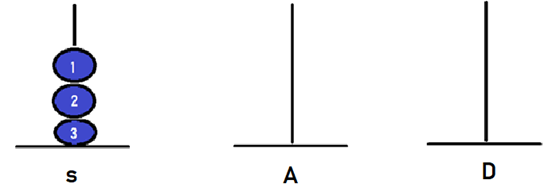Now, when i=1,(1%3==1) so there will be movement of the disk between the source(S) and destination(D) pole.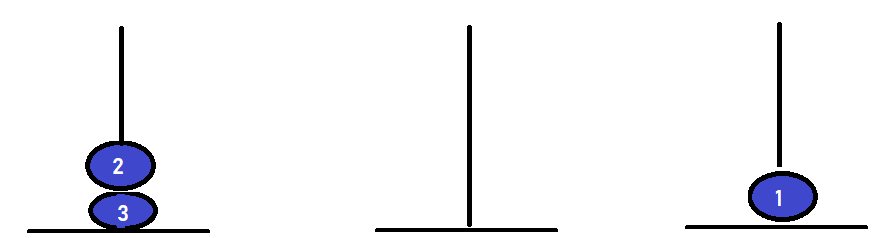Now, when i=2,(2%3==2) so there will be movement of the disk between the source(S) and auxiliary(A) pole.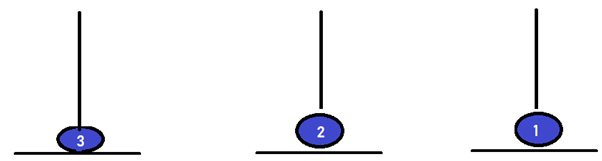Now, when i=3,(3%3==0) so there will be movement of the disk between auxiliary(A) and destination(D) pole.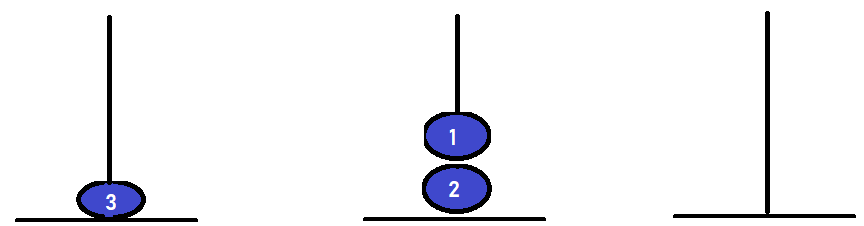Now, when i=4,(4%3==1) so there will be movement of the disk between Source(s) and destination(D) pole.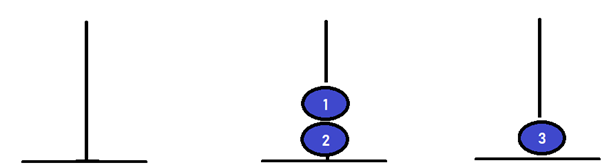Now, when i=5,(5%3==2) so there will be movement of the disk between the source(S) and auxiliary(A) pole.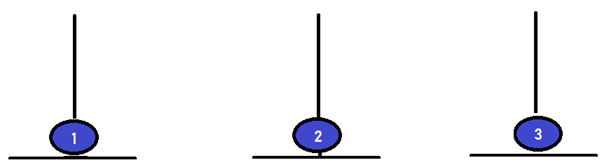Now, when i=6,(6%3==0) so there will be movement of the disk between auxiliary(A) and destination(D) pole.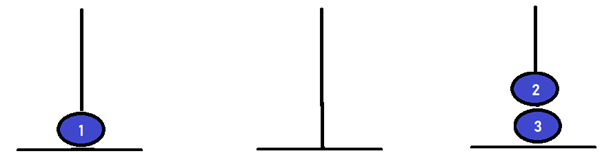Now, when i=7,(7%3==1) so there will be movement of the disk between source(S) and destination(D) pole.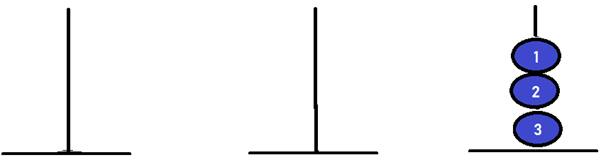Note: All these 3 disks are of different sizes. Disk named as ‘3’ being the largest and ‘1’ as the smallest.Please try to solve Tower of Hanoi on CodeStudio before stepping into the solution

## Implementation in Java

To write the iterative approach, we will use Stack Data Structure. The stack will store and remove the disks in LIFO(Last In First Order) principle.

``````import java.util.*;
import java.lang.*;
import java.io.*;

// Tower of Hanoi
class Solution {

//Stack
class Stack {
int capacity;
int top;
int arr[];
}

// Create Stack
Stack create(int capacity) {

Stack stack = new Stack();
stack.capacity = capacity;
stack.top = -1;
stack.arr = new int[capacity];

return stack;
}

//Stack is full when the top is equal
// to the last index
boolean isFull(Stack stack) {
return (stack.top == stack.capacity - 1);
}

// To check Stack is empty or not
boolean isEmpty(Stack stack) {
return (stack.top == -1);
}

//Function to add an item in Stack
void push(Stack stack, int item) {
if (isFull(stack))
return;

stack.arr[++stack.top] = item;
}

//Function to remove an item from Stack
int pop(Stack stack) {

if (isEmpty(stack))
return Integer.MIN_VALUE;

return stack.arr[stack.top--];
}

// Function to move disks between the poles
void moveDisks(Stack src, Stack dest, char s, char d) {

int pole1 = pop(src);
int pole2 = pop(dest);

// When pole 1 is empty
if (pole1 == Integer.MIN_VALUE) {

push(src, pole2);
move(d, s, pole2);
}

// When pole2 pole is empty
else if (pole2 == Integer.MIN_VALUE) {

push(dest, pole1);
move(s, d, pole1);
}

// When top disk of pole1 > top disk of pole2
else if (pole1 > pole2) {

push(src, pole1);
push(src, pole2);
move(d, s, pole2);
}
// When top disk of pole1 < top disk of pole2
else {

push(dest, pole2);
push(dest, pole1);
move(s, d, pole1);
}
}

//Function to show the movement of disks
void move(char fromPeg, char toPeg, int disk) {

System.out.println("Move the disk " + disk + " from " + fromPeg + " to " + toPeg);
}

// Implementation
void Iterative(int num, Stack src, Stack aux, Stack dest) {

int i, total_num_of_moves;
char s = 'S', d = 'D', a = 'A';

// Rules in algorithm will be followed
if (num % 2 == 0) {

char temp = d;
d = a;
a = temp;
}
total_num_of_moves = (int)(Math.pow(2, num) - 1);

// disks with large diameter are pushed first
for (i = num; i >= 1; i--)
push(src, i);

for (i = 1; i <= total_num_of_moves; i++) {

if (i % 3 == 1)
moveDisks(src, dest, s, d);

else if (i % 3 == 2)
moveDisks(src, aux, s, a);

else if (i % 3 == 0)
moveDisks(aux, dest, a, d);
}
}

// Main Function
public static void main(String[] args) {

// number of disks
int num = 3;

Solution ob = new Solution();
Stack src, dest, aux;

src = ob.create(num);
dest = ob.create(num);
aux = ob.create(num);

ob.Iterative(num, src, aux, dest);
}
}``````

Output:

``````Move the disk 1 from S to D
Move the disk 2 from S to A
Move the disk 1 from D to A
Move the disk 3 from S to D
Move the disk 1 from A to S
Move the disk 2 from A to D
Move the disk 1 from S to D``````

## Implementation in C++

``````#include <bits/stdc++.h>

using namespace std;
// Tower of Hanoi

class Stack {
public:
int capacity;
int top;
int arr;

Stack(int capacity) {
this -> capacity = capacity;
this -> top = -1;
}

//Stack is full when the top is equal
// to the last index
bool isFull(Stack * stack) {
return (stack -> top == stack -> capacity - 1);
}

// To check Stack is empty or not
bool isEmpty(Stack * stack) {
return (stack -> top == -1);
}

//Function to add an item in Stack
void push(Stack * stack, int item) {
if (isFull(stack))
return;
stack -> arr[++stack -> top] = item;
}

//Function to remove an item from Stack
int pop(Stack * stack) {
if (isEmpty(stack))
return INT_MIN;
return stack -> arr[stack -> top--];
}

void moveDisks(Stack * src, Stack * dest, char s, char d) {

int pole1 = pop(src);
int pole2 = pop(dest);

// When pole 1 is empty
if (pole1 == INT_MIN) {
push(src, pole2);
move(d, s, pole2);
}
// When pole2 pole is empty
else if (pole2 == INT_MIN) {
push(dest, pole1);
move(s, d, pole1);
}
// When top disk of pole1 > top disk of pole2
else if (pole1 > pole2) {
push(src, pole1);
push(src, pole2);
move(d, s, pole2);
}
// When top disk of pole1 < top disk of pole2
else {

push(dest, pole2);
push(dest, pole1);
move(s, d, pole1);
}
}

//Function to show the movement of disks
void move(char fromPeg, char toPeg, int disk) {
cout << "Move the disk " << disk << " from " << fromPeg << " to " << toPeg << '\n';
}

// Implementation
void Iterative(int num, Stack * src, Stack * aux, Stack * dest) {

int i, total_num_of_moves;
char s = 'S', d = 'D', a = 'A';

// Rules in algorithm will be followed
if (num % 2 == 0) {
char temp = d;
d = a;
a = temp;
}
total_num_of_moves = (int)(pow(2, num) - 1);

// disks with large diameter are pushed first
for (i = num; i >= 1; i--)
push(src, i);

for (i = 1; i <= total_num_of_moves; i++) {
if (i % 3 == 1)
moveDisks(src, dest, s, d);
else if (i % 3 == 2)
moveDisks(src, aux, s, a);
else if (i % 3 == 0)
moveDisks(aux, dest, a, d);
}
}
};

int main() {
// number of disks
int num = 3;
Stack * src, * dest, * aux;

//stacks created for src , dest, aux
src = new Stack(num);
dest = new Stack(num);
aux = new Stack(num);

// solution
Stack * sol = new Stack(0);
sol -> Iterative(num, src, aux, dest);
return 0;
}``````

Output:

``````Move the disk 1 from S to D
Move the disk 2 from S to A
Move the disk 1 from D to A
Move the disk 3 from S to D
Move the disk 1 from A to S
Move the disk 2 from A to D
Move the disk 1 from S to D``````

## Implementation in Python

``````#Tower of Hanoi
INT_MIN = -2147483648

class Stack:
def __init__(self, capacity):
self.capacity = capacity
self.top = -1
self.arr = []

# Stack is full when the top is equal
# to the last index
def isFull(self, stack):
return stack.top == stack.capacity - 1

# To check Stack is empty or not
def isEmpty(self, stack):
return stack.top == -1

# Function to add an item in Stack
def push(self, stack, item):
if self.isFull(stack):
return
stack.top+=1
stack.arr.append(item)

# Function to remove an item from Stack
def pop(self, stack):
if self.isEmpty(stack):
return INT_MIN
stack.top-=1
return stack.arr.pop()

def moveDisks(self, src, dest, s, d):

pole1 = self.pop(src);
pole2 = self.pop(dest);

# When pole 1 is empty
if(pole1 == INT_MIN):
self.push(src, pole2)
self.move(d, s, pole2)

# When pole2 pole is empty
elif (pole2 == INT_MIN):
self.push(dest, pole1)
self.move(s, d, pole1)

# When top disk of pole1 > top disk of pole2
elif (pole1 > pole2):
self.push(src, pole1)
self.push(src, pole2)
self.move(d, s, pole2)
# When top disk of pole1 < top disk of pole2
else:
self.push(dest, pole2)
self.push(dest, pole1)
self.move(s, d, pole1)

# Function to show the movement of disks
def move(self, fromPeg, toPeg, disk):
print("Move the disk "+str(disk)+" from "+fromPeg+" to " + toPeg)

# Implementation
def Iterative(self, num, src, aux, dest):

s, d, a = 'S', 'D', 'A'

# Rules in algorithm will be followed
if num % 2 == 0:
temp = d
d = a
a = temp

total_num_of_moves = int(pow(2, num) - 1)

# disks with large diameter are pushed first
i = num
while(i>=1):
self.push(src, i)
i-=1

i = 1
while(i <= total_num_of_moves):
if (i % 3 == 1):
self.moveDisks(src, dest, s, d)

elif (i % 3 == 2):
self.moveDisks(src, aux, s, a)

elif (i % 3 == 0):
self.moveDisks(aux, dest, a, d)

i+=1

# 	number of disks
num = 3
# stacks created for src , dest, aux
src  = Stack(num)
dest = Stack(num)
aux = Stack(num)
# solution
sol = Stack(0)
sol.Iterative(num, src, aux, dest)``````

Output:

``````Move the disk 1 from S to D
Move the disk 2 from S to A
Move the disk 1 from D to A
Move the disk 3 from S to D
Move the disk 1 from A to S
Move the disk 2 from A to D
Move the disk 1 from S to D``````

## Complexity Analysis

Time Complexity: The time complexity of this algorithm is O(2n), n=number of disks.

Space Complexity: The space complexity will be O(n) as we are using Stack.

After understanding the above approach, you should check out this video to know the logic behind this problem.

#### How many approaches are there to solve the Tower of Hanoi problem?

There are two approaches to solve this problem:

• Iterative solution.
• Recursive solution.

#### What do you mean by Stack Data Structure?

It is a linear data structure that stores and removes elements based on LIFO(Last In First Order) principle. There are some common operations that are implemented on stack like Push(), Peek(), Pop(), IsEmpty() etc.

#### What is the difference between iterative and recursive algorithms?

Iterative:

• It uses loop statements such as for loop or while loop to repeat the steps.
• It is faster than the recursive algorithm because of overheads.
• More efficient in terms of time and space.

Recursive:

• The Function calls itself again and again till the given base condition is satisfied.
• Less efficient in terms of time and space.

## Conclusion

In this blog, we discussed the Tower of Hanoi problem and how we can build an approach for it:

There are two approaches for this approach; we have discussed the iterative solution in which we have used the Stack to store and remove the disks and arrange them in ascending order. We have written code in Java language.

You can use CodeStudio to practice various DSA questions typically asked in interviews. It will help you in mastering efficient coding techniques.

Keep Coding!!!